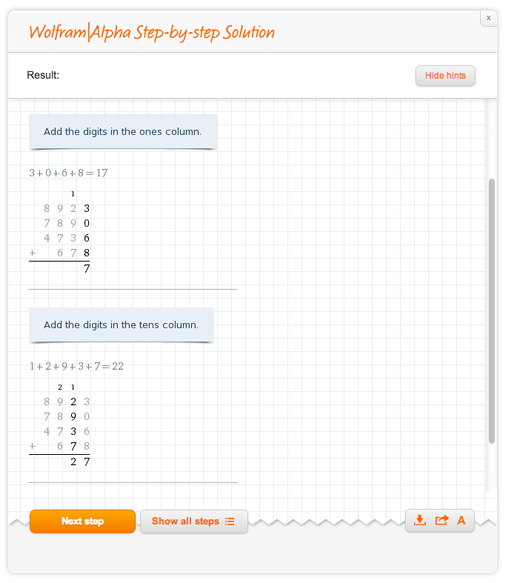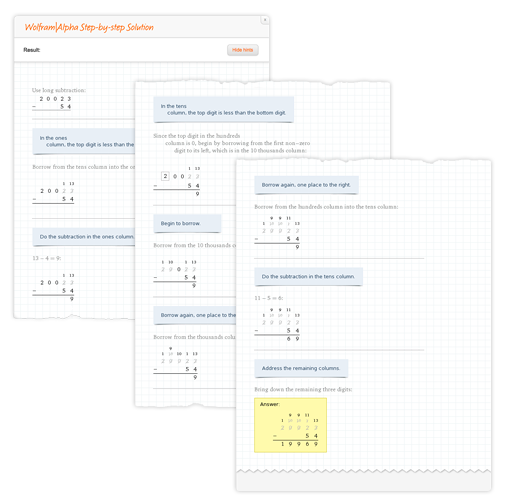# Solving Basic Arithmetic Step by Step with Wolfram|Alpha

January 28, 2013 —Comments Off

As a continuation of our new math content blog series, I’d like to talk about an exciting new Step-by-step feature. Previously I talked about differential equations, but today I’d like to look toward the other end of the spectrum: basic arithmetic. Wolfram|Alpha can now help you work out long addition, subtraction, multiplication, and division with hints and steps! Let’s go ahead and look at some examples.

A basic long addition problem such as 1234 + 3456 has few carries. When clicking the Step-by-step solution button, you’ll notice how we start at the least significant digit and work our way left, carrying when necessary. Here’s a tougher problem, 8923 + 7890 + 4736 + 678, which has more terms and carries:We can also do subtraction problems. For example, 4539 – 2356, which seems as simple as addition. Problems can get a bit trickier in some cases though. Borrowing from zero is one such case, as shown with 20023 – 54:Getting a bit more advanced, let’s look some multiplication examples starting with 113 x 23. Each time we multiply digits, there is a chance it will be greater than 9. In these cases, we must carry. Let’s look at 2308 x 53:

Lastly we have division, which is the toughest out of these four. We’ll use 12402 / 53 as an example: